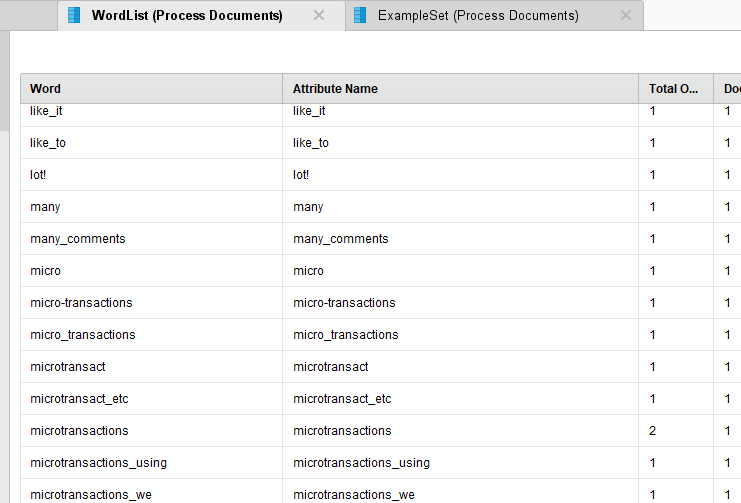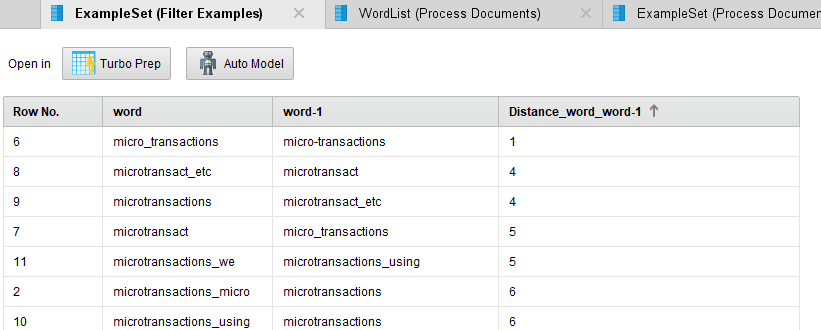Member Posts: 13Contributor I
Hello there!

We are currently writing a research project on microtransactions using natural language processing.

We have a Excel file containing 450.000 comments.

As to capture as many comments related to microtransactions, we would like to concatenate som variations of the spelling e.g.

Microtransactions = "micro transactions", "micro-transactions", "microtransact" etc...

We would very much like it to return all the 450.000 comments, though with the words concatenated as explained above.

How do we best achieve this?

Thanks a lot!

• Moderator, RapidMiner Certified Analyst, RapidMiner Certified Expert, Member Posts: 1,635Unicorn
After you tokenize your text (using non-letter mode), there are RapidMiner stemming operators that can be used to combine tokens based on word roots using various dictionaries built for this purpose (Porter, snowball, etc.).  But if your words are uncommon then you may need to supplement with your own replacement dictionary (which is also supported in RapidMiner).  I would probably start with a sample of your corpus, see how the standard stemmers work, and then see what you may still need to add.
Brian T.
Lindon Ventures
Data Science Consulting from Certified RapidMiner Experts
• Administrator, Employee, RapidMiner Certified Analyst, RapidMiner Certified Expert, Member Posts: 363RM Data Scientist
edited February 2019
Hi @mavi16ab,

Have you tried the Levenshtein Distance from operator toolbox extension? This could help you find the similar strings.

Suppose you have processed the 450000 comments with tokenize inside text mining operators, like "process documents", you will get a wordlist like thisThen you convert wordlist to data and generate pairs of keywords then apply the levenshtein distance on the pair-wised keywords.
I did a lagging on wordlist for a quick demo. But for n keywords, you will basically need n*(n-1)/2 pairs of keywords for distance calculation. Data to similarity operator will help you to expand data into pairwised format in a quick way.```<?xml version="1.0" encoding="UTF-8"?><process version="9.2.000">
<context>
<input/>
<output/>
<macros/>
</context>
<operator activated="true" class="process" compatibility="9.2.000" expanded="true" name="Process">
<parameter key="logverbosity" value="init"/>
<parameter key="random_seed" value="2001"/>
<parameter key="send_mail" value="never"/>
<parameter key="process_duration_for_mail" value="30"/>
<parameter key="encoding" value="SYSTEM"/>
<process expanded="true">
<operator activated="true" class="text:create_document" compatibility="8.1.000" expanded="true" height="68" name="Create Document" width="90" x="45" y="34">
<parameter key="text" value="Hello there!&#10;&#10;We are currently writing a research project on microtransactions using natural language processing.&#10;&#10;We have a Excel file containing 450.000 comments. &#10;&#10;As to capture as many comments related to microtransactions, we would like to concatenate som variations of the spelling e.g.&#10;&#10;Microtransactions = &quot;micro transactions&quot;, &quot;micro-transactions&quot;, &quot;microtransact&quot; etc...&#10;&#10;We would very much like it to return all the 450.000 comments, though with the words concatenated as explained above.&#10;&#10;How do we best achieve this?&#10;&#10;Thanks a lot!"/>
<parameter key="label_type" value="nominal"/>
</operator>
<operator activated="true" class="text:process_documents" compatibility="8.1.000" expanded="true" height="103" name="Process Documents" width="90" x="179" y="34">
<parameter key="create_word_vector" value="true"/>
<parameter key="vector_creation" value="TF-IDF"/>
<parameter key="keep_text" value="false"/>
<parameter key="prune_method" value="none"/>
<parameter key="prune_below_percent" value="3.0"/>
<parameter key="prune_above_percent" value="30.0"/>
<parameter key="prune_below_rank" value="0.05"/>
<parameter key="prune_above_rank" value="0.95"/>
<parameter key="datamanagement" value="double_sparse_array"/>
<parameter key="data_management" value="auto"/>
<process expanded="true">
<operator activated="true" class="text:tokenize" compatibility="8.1.000" expanded="true" height="68" name="Tokenize" width="90" x="112" y="34">
<parameter key="mode" value="specify characters"/>
<parameter key="characters" value=",.: &quot;=_"/>
<parameter key="expression" value=" \.\&quot;\-"/>
<parameter key="language" value="English"/>
<parameter key="max_token_length" value="3"/>
</operator>
<operator activated="true" class="text:transform_cases" compatibility="8.1.000" expanded="true" height="68" name="Transform Cases" width="90" x="246" y="34">
<parameter key="transform_to" value="lower case"/>
</operator>
<operator activated="true" class="text:generate_n_grams_terms" compatibility="8.1.000" expanded="true" height="68" name="Generate n-Grams (Terms)" width="90" x="380" y="34">
<parameter key="max_length" value="2"/>
</operator>
<operator activated="true" class="text:filter_by_length" compatibility="8.1.000" expanded="true" height="68" name="Filter Tokens (by Length)" width="90" x="581" y="34">
<parameter key="min_chars" value="4"/>
<parameter key="max_chars" value="25"/>
</operator>
<connect from_port="document" to_op="Tokenize" to_port="document"/>
<connect from_op="Tokenize" from_port="document" to_op="Transform Cases" to_port="document"/>
<connect from_op="Transform Cases" from_port="document" to_op="Generate n-Grams (Terms)" to_port="document"/>
<connect from_op="Generate n-Grams (Terms)" from_port="document" to_op="Filter Tokens (by Length)" to_port="document"/>
<connect from_op="Filter Tokens (by Length)" from_port="document" to_port="document 1"/>
<portSpacing port="source_document" spacing="0"/>
<portSpacing port="sink_document 1" spacing="0"/>
<portSpacing port="sink_document 2" spacing="0"/>
</process>
</operator>
<operator activated="true" class="text:wordlist_to_data" compatibility="8.1.000" expanded="true" height="82" name="WordList to Data" width="90" x="313" y="85"/>
<operator activated="true" class="select_attributes" compatibility="9.2.000" expanded="true" height="82" name="Select Attributes" width="90" x="447" y="187">
<parameter key="attribute_filter_type" value="subset"/>
<parameter key="attribute" value=""/>
<parameter key="attributes" value="word"/>
<parameter key="use_except_expression" value="false"/>
<parameter key="value_type" value="attribute_value"/>
<parameter key="use_value_type_exception" value="false"/>
<parameter key="except_value_type" value="time"/>
<parameter key="block_type" value="attribute_block"/>
<parameter key="use_block_type_exception" value="false"/>
<parameter key="except_block_type" value="value_matrix_row_start"/>
<parameter key="invert_selection" value="false"/>
<parameter key="include_special_attributes" value="false"/>
</operator>
<operator activated="true" class="data_to_similarity" compatibility="9.2.000" expanded="true" height="82" name="Data to Similarity" width="90" x="581" y="187">
<parameter key="measure_types" value="MixedMeasures"/>
<parameter key="mixed_measure" value="MixedEuclideanDistance"/>
<parameter key="nominal_measure" value="NominalDistance"/>
<parameter key="numerical_measure" value="EuclideanDistance"/>
<parameter key="divergence" value="GeneralizedIDivergence"/>
<parameter key="kernel_gamma" value="1.0"/>
<parameter key="kernel_sigma1" value="1.0"/>
<parameter key="kernel_sigma2" value="0.0"/>
<parameter key="kernel_sigma3" value="2.0"/>
<parameter key="kernel_degree" value="3.0"/>
<parameter key="kernel_shift" value="1.0"/>
<parameter key="kernel_a" value="1.0"/>
<parameter key="kernel_b" value="0.0"/>
<description align="center" color="transparent" colored="false" width="126">use this to quickly generate pairs of keywords, you need to join by ID to get the tokens back</description>
</operator>
<operator activated="true" class="time_series:lag_series" compatibility="9.2.000" expanded="true" height="82" name="Lag" width="90" x="715" y="340">
<list key="attributes">
<parameter key="word" value="1"/>
</list>
<parameter key="overwrite_attributes" value="false"/>
<parameter key="extend_exampleset" value="false"/>
</operator>
<operator activated="true" class="operator_toolbox:levenshtein_distance" compatibility="1.7.000" expanded="true" height="82" name="Generate Levenshtein Distance" width="90" x="849" y="340">
<parameter key="first_attribute_for_distance_calculation" value="word"/>
<parameter key="second_attribute_for_distance_calculation" value="word-1"/>
</operator>
<operator activated="true" class="filter_examples" compatibility="9.2.000" expanded="true" height="103" name="Filter Examples" width="90" x="983" y="340">
<parameter key="parameter_expression" value=""/>
<parameter key="condition_class" value="custom_filters"/>
<parameter key="invert_filter" value="false"/>
<list key="filters_list">
<parameter key="filters_entry_key" value="word.contains.micro"/>
</list>
<parameter key="filters_logic_and" value="true"/>
</operator>
<connect from_op="Create Document" from_port="output" to_op="Process Documents" to_port="documents 1"/>
<connect from_op="Process Documents" from_port="example set" to_port="result 1"/>
<connect from_op="Process Documents" from_port="word list" to_op="WordList to Data" to_port="word list"/>
<connect from_op="WordList to Data" from_port="word list" to_port="result 2"/>
<connect from_op="WordList to Data" from_port="example set" to_op="Select Attributes" to_port="example set input"/>
<connect from_op="Select Attributes" from_port="example set output" to_op="Data to Similarity" to_port="example set"/>
<connect from_op="Data to Similarity" from_port="example set" to_op="Lag" to_port="example set input"/>
<connect from_op="Lag" from_port="example set output" to_op="Generate Levenshtein Distance" to_port="exa"/>
<connect from_op="Generate Levenshtein Distance" from_port="out" to_op="Filter Examples" to_port="example set input"/>
<connect from_op="Filter Examples" from_port="example set output" to_port="result 3"/>
<portSpacing port="source_input 1" spacing="0"/>
<portSpacing port="sink_result 1" spacing="0"/>
<portSpacing port="sink_result 2" spacing="0"/>
<portSpacing port="sink_result 3" spacing="252"/>
<portSpacing port="sink_result 4" spacing="0"/>
</process>
</operator>
</process>
```

• Member Posts: 1Newbie
Have you tried the Levenshtein Distance from operator toolbox extension? This could help you find the similar strings.